# Exponential equation

Find x, if 625 ^ x = 5

The equation is exponential because the unknown is in the exponential power of 625

Result

x =  0.25

#### Solution:Leave us a comment of example and its solution (i.e. if it is still somewhat unclear...):

Showing 0 comments:Be the first to comment!#### To solve this example are needed these knowledge from mathematics:

Do you have a linear equation or system of equations and looking for its solution? Or do you have quadratic equation?

## Next similar examples:

1. Geometric progressionIn geometric progression, a1 = 7, q = 5. Find the condition for n to sum first n members is: sn≤217.
2. Exp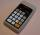If ?, then n is:
3. Powers 3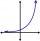2 to the power of n divided by 4 to the power of -3 equal 4. What is the vaule of n?
4. Exponential equationSolve for x: (4^x):0,5=2/64.
5. Water lilies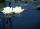Water lilies are growing on the pond and their number is doubled every day. The whole layer is covered in 12 days. How many days will it cover 8 layers?
6. The city 3The city has 22,000 residents. How long it is expected to have 25,000 residents if the average annual population growth is 1.4%?
7. Exponential equationIn the set R solve the equation: ?
8. Logif ?, what is b?
9. SequenceCalculate what member of the sequence specified by ? has value 86.
10. Computer revolution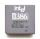When we started playing with computers, the first processor, which I remember was the Intel 8080 from 1974, with the performance of 0.5 MIPS. Calculate how much percent a year rose CPU performance when Intel 486DX from 1992 has 54 MIPS. What
11. Exponential equationSolve exponential equation (in real numbers): 98x-2=9
12. Coordinate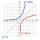Determine missing coordinate of the point M [x, 120] of the graph of the function f bv rule: y = 5x
13. LogarithmDetermine the number whose decimal logarithm is -3.8.
14. Solve equationsolve equation: ?
15. Intercept with axisF(x)=log(x+4)-2, what is the x intercept
16. CalculationHow much is sum of square root of six and the square root of 225?
17. Theorem proveWe want to prove the sentense: If the natural number n is divisible by six, then n is divisible by three. From what assumption we started?